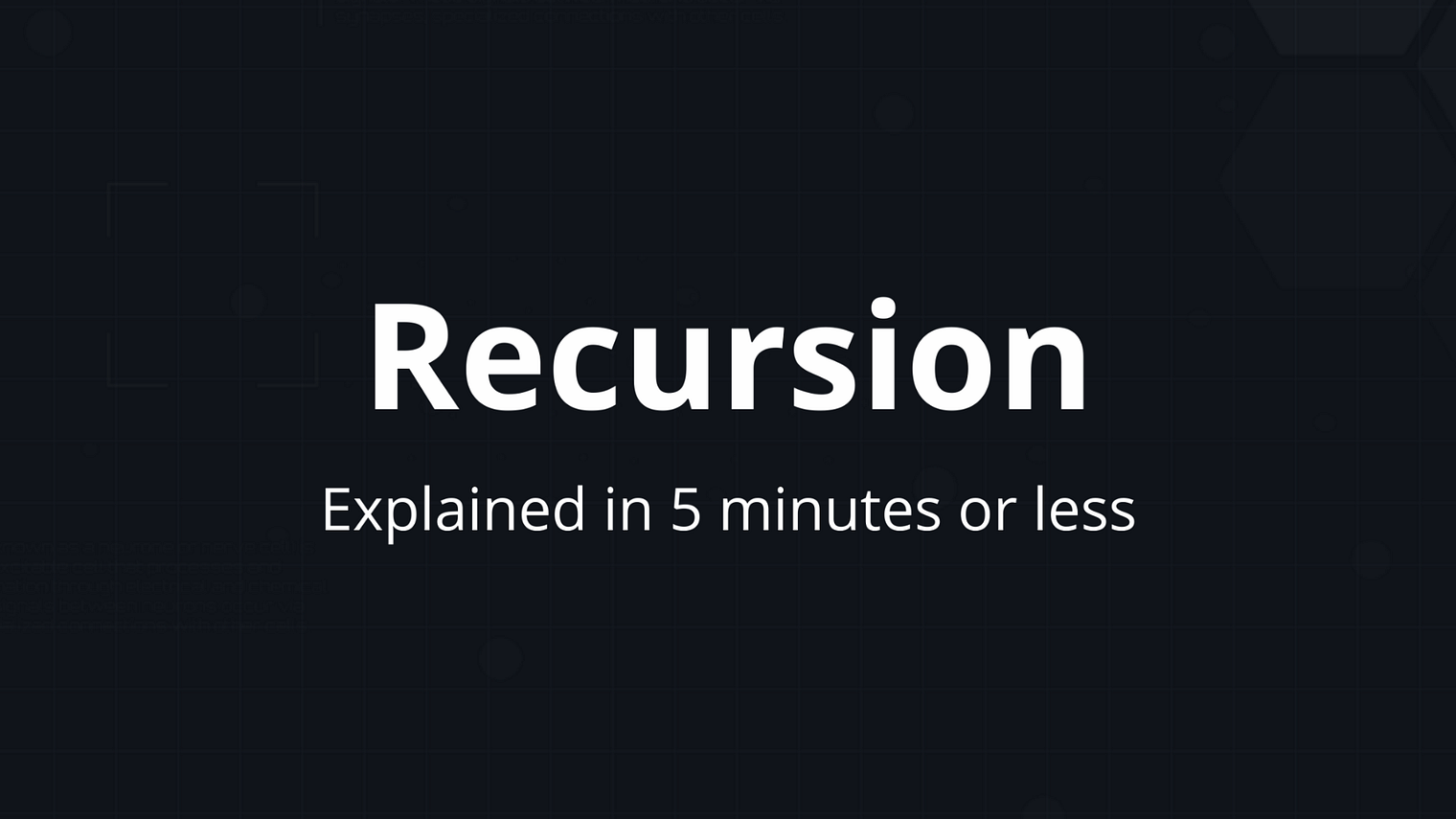# What is Recursion?## What is Recursion?

Recursion simply means “self reference”. When something refers to itself or describes itself, it is called recursive.

For example > “This sentence is recursive.”

is recursive.

This picture is also recursive:In programming, recursive function is a function that calls itself

Let’s say you and your friends have ordered pizza, and now you want to cut it into equal slices. To do that you cut it in half, then you cut the resulting slices in half, and keep going until there’s enough slices for everyone.

In code, it would look like this:

```def slice_pizza(number_of_friends, number_of_slices):
# Cut pizza in half
number_of_slices = number_of_slices*2

# Is there enough slices for everybody?
if number_of_slices &gt;= number_of_friends:
# If yes - return our slices, it's time to eat pizza!
return number_of_slices
else:
# If not - then cut it in half once again.
return slice_pizza(number_of_friends, number_of_slices)

print(slice_pizza(15, 1))
```

This function takes the number of friends in the room(15), and a number of slices of pizza(initially 1). It cuts pizza in half:

```number_of_slices = number_of_slices*2
```

and checks if there’s enough of the slices now. If yes — it just returns the number of resulting slices, if not — it calls itself again, to cut the pizza in half one more time.

Recursive functions can be hard to think about, but there’s a whole class of problems in Computer Science that are much easier solved by using this kind of algorithms. For example it can be used for binary search, for finding a factorial of a number, or for creating a system of nested comments like on reddit.

Receive weekly digest of my best posts!